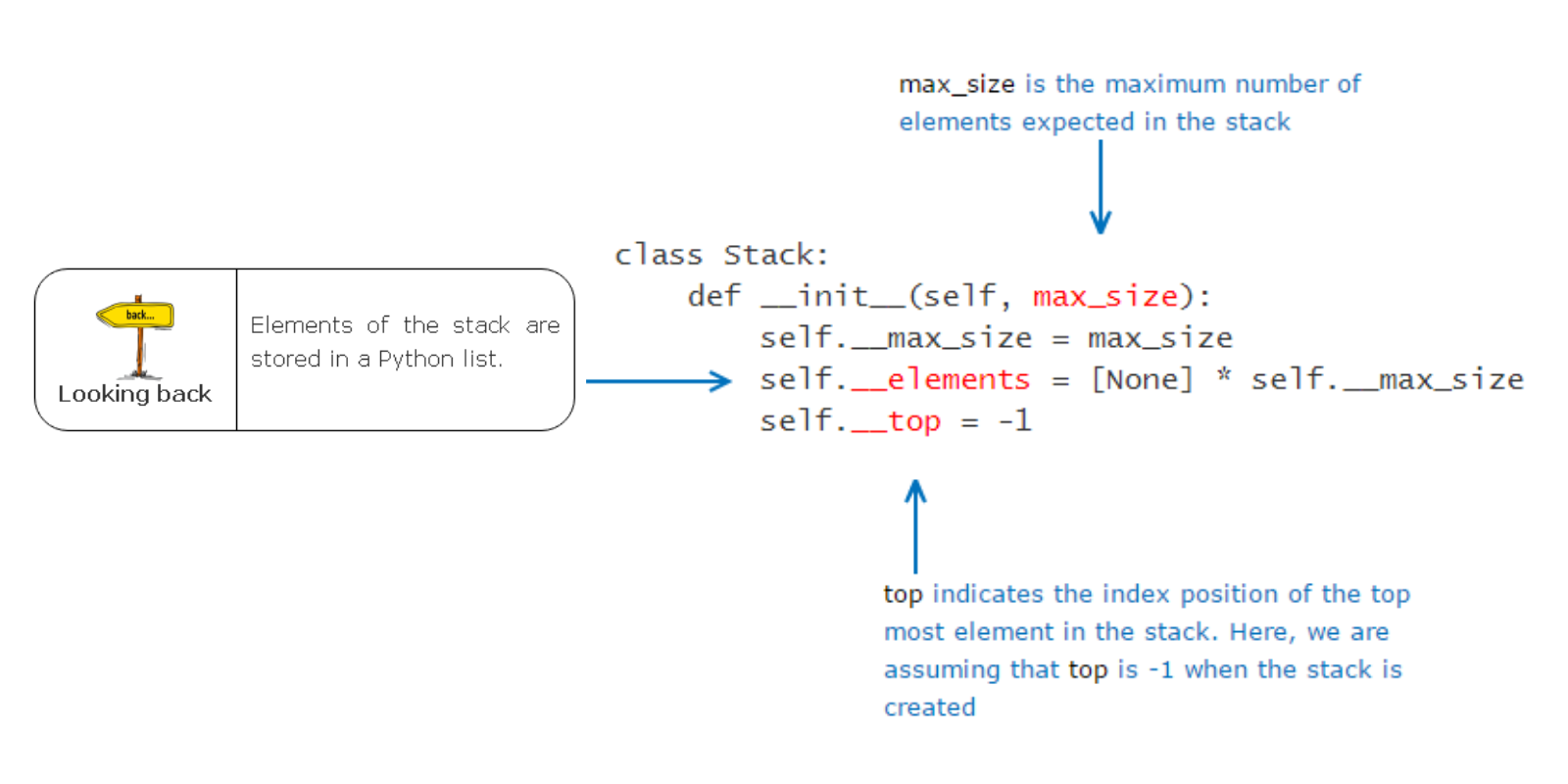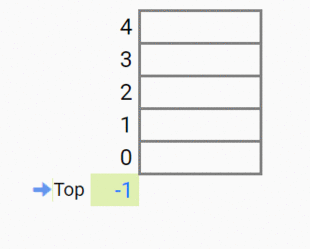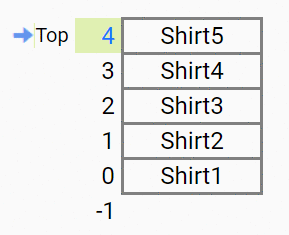# Data Structures and Algorithms using Python (Part 5 : Stack)

Data Structures DATA STRUCTURES AND ALGORITHMS USING PYTHON SERIESTill the last part, we had completed Lists and Linked Lists. In this part, we are going to learn about another data structure called Stack.
Consider this situation :

Sophia is arranging the shirts of David one on top of the other in the cupboard. She is very particular that David should always wear the shirt at the top.Do you think David can take the shirts in any order? If he does, Sophia’s hard work will go in vain and the shirts will not be arranged now as they were before.
This pile of shirts arranged one on top of the other is known as Stack. It follows Last-In-First-Out (LIFO) or First-In-Last-Out (FILO) principle. If we consider the above situation, David can take the shirt which is on the top if he doesn’t wish to ruin the arrangement, i.e, the shirt which was put at the last will be taken out first. This means whatever operation we operate on stack, it will be done on the top most element itself.
Thus we have the following two operations on a stack :

1. Push or insert an element to the top of the stack
2. Pop or remove an element from top of the stack

Let’s have a class Stack as follows:### Push Operation

Observe this :Initially, top is pointing to -1. When Shirt1 is to be inserted, top is incremented by 1 and Shirt1 is inserted. Similarly, when Shirt2 is to be inserted, top is incremented by 1 and Shirt2 is inserted.
Let’s look at the algorithm closely :

#### Algorithm

``````push(data):
1. Check whether the stack is full. If full, display appropriate message
2. If not,
a. increment top by one
b. Add the element at top position in the elements array``````

Let’s implement the above algorithm in the code. We need a Stack Class having two methods : push(data) to insert data and is_full() to check if the stack is already full.

``````class Stack:
def __init__(self,max_size):
self.__max_size=max_size
self.__elements=[None]*self.__max_size
self.__top=-1

def get_max_size(self):
return self.__max_size

def is_full(self):
if(self.__top==self.__max_size-1):
return True
return False

def push(self,data):
if(self.is_full()):
print("The stack is full!!")
else:
self.__top+=1
self.__elements[self.__top]=data

#You can use the below __str__() to print the elements of the DS object while debugging
def __str__(self):
msg=[]
index=self.__top
while(index>=0):
msg.append((str)(self.__elements[index]))
index-=1
msg=" ".join(msg)
msg="Stack data(Top to Bottom): "+msg
return msg

stack1=Stack(5)
#Push all the required element(s).
stack1.push("Shirt1")
stack1.push("Shirt2")
print(str(stack1))``````

In the above code, we have a Stack class with the above mentioned methods. Let’s understand how it works. First we initialize an object of the Stack class with the max_size parameter which decides the maximum number of elements that can be inserted into the stack. Along with this, an empty list of length max_size is initialized with top pointing to -1.
We then call the push method to insert Shirt1 into our stack. Push method first checks if the stack is already full using the is_full() method. If it is, an appropriate error is thrown else top is incremented by 1 and the element is inserted at the index at which top is pointing.
This is how push operation occurs within a stack.

### Pop Operation

Observe this :Initially, our stack is full and top is pointing to the top-most element. First the data located at the index at which top is pointing, is removed (or retrieved) and then top is decremented by 1. This is how pop operation works.
Let’s look at its algorithm :

#### Algorithm

``````pop:
1. Check whether the stack is empty. If empty, display appropriate message
2. If not,
a. Retrieve data at the top of the stack
b. Decrement top by 1
c. Return the retrieved data``````

Let’s implement the above algorithm in the code. For the pop operation, we’re going to use is_empty() method to check if the stack is empty and pop() method to remove top-most element. Note that, for the pop operation we don’t need to pass any element or index as argument since it will always operate on the top-most element.

``````class Stack:
def __init__(self,max_size):
self.__max_size=max_size
self.__elements=[None]*self.__max_size
self.__top=-1

def get_max_size(self):
return self.__max_size

def is_full(self):
if(self.__top==self.__max_size-1):
return True
return False

def push(self,data):
if(self.is_full()):
print("The stack is full!!")
else:
self.__top+=1
self.__elements[self.__top]=data

def is_empty(self):
if(self.__top==-1):
return True
return False

def pop(self):
if(self.is_empty()):
print("The stack is empty!!")
else:
data= self.__elements[self.__top]
self.__top-=1
return data

def display(self):
if(self.is_empty()):
print("The stack is empty")
else:
index=self.__top
while(index>=0):
print(self.__elements[index])
index-=1

#You can use the below __str__() to print the elements of the DS object while debugging
def __str__(self):
msg=[]
index=self.__top
while(index>=0):
msg.append((str)(self.__elements[index]))
index-=1
msg=" ".join(msg)
msg="Stack data(Top to Bottom): "+msg
return msg

stack1=Stack(5)
#Push all the required element(s).
stack1.push("Shirt1")
stack1.push("Shirt2")
stack1.push("Shirt3")
stack1.push("Shirt4")
stack1.push("Shirt5")
#Pop an element
popped = stack1.pop()
print(f"Element popped is {popped}")
stack1.display()``````

For the pop operation, we first checked if the stack is already empty using the is_empty() method. If it is, we throw an appropriate error, else we first retrieve the data at the index top is pointing as of now. Then, top is decremented by 1. If we wish to, we can return the data that we’ve retrieved.

We’ve an additional display() method to print the elements in the stack. It first checks if the stack is empty. If it isn’t, we start iterating the stack from the index of the top-most element until the index becomes 0.

### Applications of Stack

This data structure has some important applications in different aspect.

1. Infix to Postfix or Infix to Prefix Conversion : The stack can be used to convert some infix expression into its postfix equivalent, or prefix equivalent. These postfix or prefix notations are used in computers to express some expressions. These expressions are not so much familiar to the infix expression, but they have some great advantages also. We do not need to maintain operator ordering, and parenthesis.
2. Postfix or Prefix Evaluation : After converting into prefix or postfix notations, we have to evaluate the expression to get the result. For that purpose also, we need the help of stack data structure.

3. Another great use of stack is during the function call and return process. When we call a function from one other function, that function call statement may not be the first statement. After calling the function, we also have to come back from the function area to the place, where we have left our control. So we want to resume our task, not restart. For that reason, we store the address of the program counter into the stack, then go to the function body to execute it. After completion of the execution, it pops out the address from stack and assign it into the program counter to resume the task again.

### Undo-Redo in Clipboard

Clipboard in Windows uses two stacks to implement Undo (Ctrl+Z) and Re-do (Ctrl+Y) operations. Let’s understand this :

You would have worked on Windows word editors such as MS-Word, Notepad etc. Here is a text written in MS-Word. Observe how the text changed on click of Ctrl-Z and Ctrl-Y.Here is how undo-redo operations are implemented for the example discussed.### Conclusion

In this part, we’ve learnt about Stacks, operations on stacks and its applications. In the upcoming articles, other data structures like Queues and Graphs will be discussed.
You can also read my blogs on Medium as well.

Stay Tuned, thanks!

Tags: #Coding, #Python